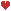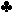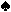9,2,3,3,Q +K,A = 1.03141312 = One Pair of Threes10,J = 1.03121110 = One Pair of Threes10,3 = 3.031210 = Three Of A Kind - ThreesJ,2 = 2.030212 = Two Pairs - Threes and Twos4,Q = 2.120309 = Two Pairs - Queens and Threes9,Q = 2.120903 = Two Pairs - Queens and Nines6,4 = 1.03120906 = One Pair of Threes5,7 = 1.03120907 = One Pair of ThreesJ,7 = 1.03121109 = One Pair of Threes8,9 = 2.090312 = Two Pairs - Nines and ThreesK,8 = 1.03131209 = One Pair of Threes5,2 = 2.030212 = Two Pairs - Threes and Twos Winner: Three Of A Kind - Threes (3.031210)

0.0002

3.03121